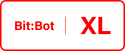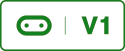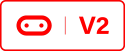# Path Finding Robot (Arduino IDE)Here we will use the HC-SR04 Ultrasonic sensor to give Bot:Bot eyes. These sensors can be purchased on the internet and I suggest you buy one from 4Tronix as they have mounted it on a breakout board to get over the voltage issues (the sensor runs on 5v logic where as the Micro:Bit runs on 3v logic).

I have created a simple path finding algorithm as an introduction to this type of programming. One of the problems that we encounter is that if we explicitly tell Bit:bot what to do every time it encounters an obstacle it will move in a predictable way that will mean that the robot will either get stuck or go around in circles in certain scenarios. To combat this we will use random delays when the robot turns and random number of turns before changing direction.

I opened up the previous project and created a new tab called _03_PathFinder and added the following code;

A Simple Path Finder
``/* * Path finding algorithm for the 4Tronix Bit:Bot * Author David Bradshaw 2018 * Requires the ultrasonic sensor HC-SR04 */int leftCount = 0;int rightCount = 0;boolean isLeft = true;long randDelay;long rndSide = random(512, 2048);int numberofTurns = (int(rndSide) / 100); //Will decide how many times to turn each way before changing direction/* * This algorithm will make the robot go forwards until an object is less than 25cm away * Then it will turn either left or right for a random amount of times * When it has turned either left or right for a random number or turns it will * turn the other way and the process is repeated. If an obstacle is less than 5cm * away it will go backwards for 250mS *  * This is a random pathfinder with no memory, it uses random intervals to * minimise the risk of getting stuck or going the same way over and over again. * The robots behaviour should appear to be random. */void simplePF(){    int distance = getDistance(); //Uses the ultrasonic sensor to detect obstacles    randomSeed((detectLight("LEFT") * digitalRead(rightLineSensor) * distance));    randDelay = random(512, 1024);  //Generate a random number to calculate the delay    randDelay = (randDelay / 4);        if (distance > 50)    {        setColourLeft(0, 64, 0);        setColourRight(0, 64, 0);        forwards(255);  //full speed    }    else if (distance < 50 && distance > 25)    {        setColourLeft(64, 64, 25);        setColourRight(64, 64, 25);        forwards(128);  //half speed    }    else if (distance < 25 && distance > 5)    {        //Object in front so we better turn        if(isLeft == true)        {            setColourLeft(0, 64, 0);            setColourRight(64, 0, 0);            leftCount = leftCount + 1;            left(128);            delay(randDelay);            if (leftCount > numberofTurns)            {                leftCount = 0;                isLeft = false;            }           }        else        {            setColourLeft(64, 0, 0);            setColourRight(0, 64, 0);            rightCount = rightCount + 1;            right(128);            delay(randDelay);              if (rightCount > numberofTurns)            {                rightCount = 0;                isLeft = true;            }           }    }    else if (distance < 5)    {        setColourLeft(64, 0, 0);        setColourRight(64, 0, 0);        backwards(255);        delay(250);    }}``

I then added the following line to the loop() method in the _00_programLoop tab;

Call the above Function
``/** PUT YOUR MAIN LOOP CODE HERE*/simplePF();``

Remember that your Neopixels won’t work unless you carry out the Neopixel modification and you must add all libraries if you haven’t already links are in the code.

You can download the code by clicking Bitbot_Lib_PathFinder or from the resources page.